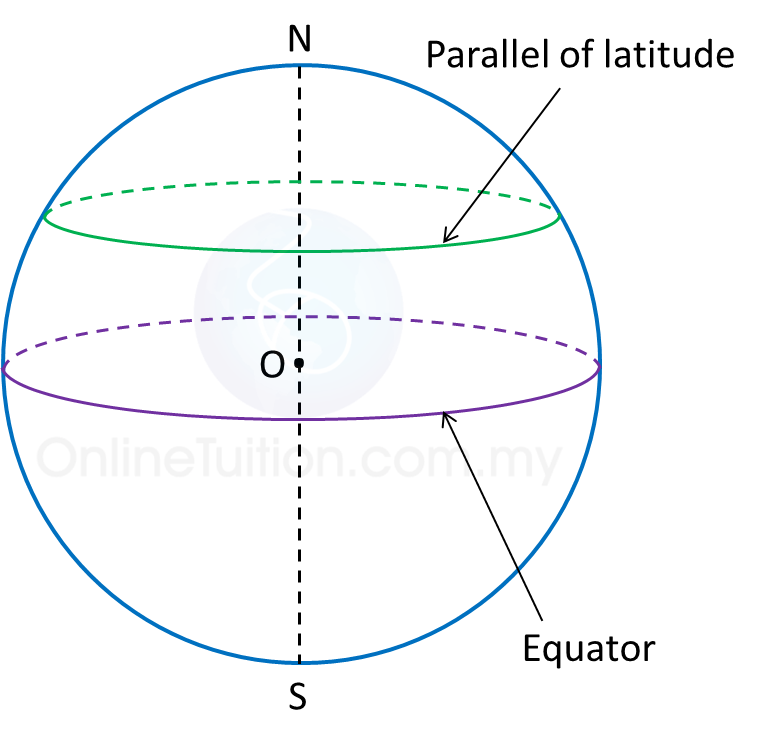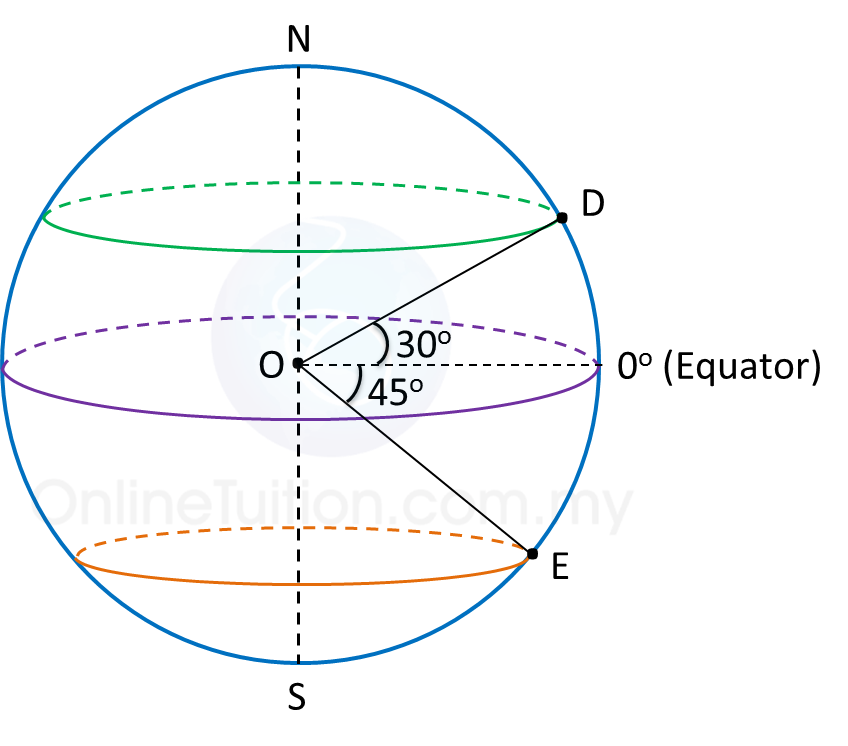# 9.2 Latitudes

9.2 Latitudes

1. The great circle which is perpendicular to the earth’s axis is called equator.2. A latitude is the angle at the centre of the Earth which is subtended by the arc of a meridian starting from the Equator to the parallel of latitude.

3. A circle which is perpendicular to the Earth’s axis and parallel to the equator is called a parallel of latitude.

4. The latitude of the Equator is 0o.

5. All points on a parallel of latitude have the same latitude.

Example:Latitude of is 30o N.
Latitude of is 45o S.

Difference between Two Latitudes
1. If both parallels of latitude are on the north (or south) of the equator, subtract the angles of latitudes.
2. If both parallels of latitude are on the opposite sides of the equator, add the angles of the latitudes.